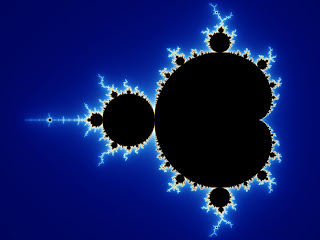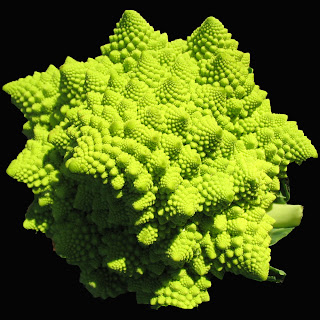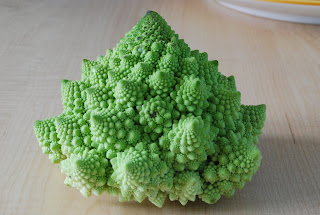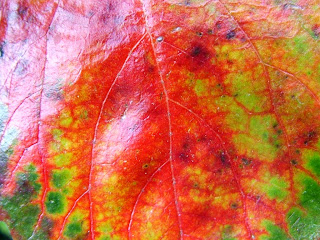## Saturday, October 24, 2009

### FractalsA fractal is "a rough or fragmented geometric shape that can be split into parts, each of which is (at least approximately) a reduced-size copy of the whole," a property called self-similarity. Roots of mathematical interest in fractals can be traced back to the late 19th Century; however, the term "fractal" was coined by Benoît Mandelbrot in 1975 and was derived from the Latinfractus meaning "broken" or "fractured." A mathematical fractal is based on an equation that undergoes iteration, a form of feedback based on

A fractal often has the following features:

Because they appear similar at all levels of magnification, fractals are often considered to be infinitely complex (in informal terms). Natural objects that approximate fractals to a degree include clouds, mountain ranges, lightning bolts, coastlines, snow flakes, various vegetables (cauliflower and broccoli), and animal coloration patterns. However, not all self-similar objects are fractals—for example, the real line (a straight Euclidean line) is formally self-similar but fails to have other fractal characteristics; for instance, it is regular enough to be described in Euclidean terms.

Images of fractals can be created using fractal-generating software. Images produced by such software are normally referred to as being fractals even if they do not have the above characteristics, as it is possible to zoom into a region of the image that does not exhibit any fractal properties.